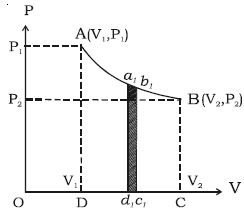# Define and Describe on Indicator Diagram or (P-V Diagram)

A curve showing variation of volume of a substance taken along the X-axis and the variation of pressure taken along Y-axis is called an indicator diagram or P-V diagram. The shape of the indicator diagram shall depend on the nature of the thermodynamical process the system undergoes.

Let consider one mole of an ideal gas enclosed in a cylinder fitted with a perfectly frictionless piston. Let P1, V1 and T be the initial state of the gas. If dV is an infinitesimally small increase in volume of the gas during which the pressure P is assumed to be constant, then small amount of workdone by the gas is, dW = PdVIn the indicator diagram, dW = area a1b1c1d1

The total workdone by the gas during expansion from V1 to V2 is

W = v2v1 PdV = area ABCD, in the indicator diagram.

Hence, in an indicator diagram the area under the curve represents the work done (Figure).